# MCQs on Electric Circuits

##### Page 40 of 63. Go to page 1 2 3 4 5 6 7 8 9 10 11 12 13 14 15 16 17 18 19 20 21 22 23 24 25 26 27 28 29 30 31 32 33 34 35 36 37 38 39 40 41 42 43 44 45 46 47 48 49 50 51 52 53 54 55 56 57 58 59 60 61 62 63
01․ What will be the rms value of rectangular wave with amplitude 10 V?
5√2 V.
10 V.
11.2 V.
7.7 V.

RMS value means, root mean square value of a wave. A rectangular voltage wave has constant amplitude in both positive and negative direction. Hence the mean value and its amplitude will be identical. Therefore square root of square of the mean value of amplitude is same as amplitude of the wave.

02․ The rms value of sinusoidal voltage wave V = 200sinω0t
100√2 V.
200 V.
100 V.
200√2 V.

The peak value of given voltage wave is 200 V.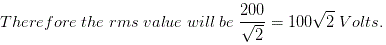03․ If one cycle of AC waveform occurs every millisecond, the frequency will be
100 Hz.
1000 Hz.
50 Hz.
10 kHz.

One cycle of AC waveform occurs every millisecond means 1000 cycles of that waveform occur in one second. The numbers of cycles of waveform per second is the frequency of that waveform.

04․ If emf in a given circuit is given by e = 100 sinω0t, then maximum value of voltage and frequency will be
100 V, 100 Hz.
100 V, 50 Hz.
100√2 V, 100 Hz.
50√2 V, 100 Hz.

The emf equation is e = 100 sinω0t ........... (1) Again the general form of emf equation is e = Vmsin2πft ........... (2) Where, Vm is the voltage amplitude and f is the frequency. Comparing equations (1) and (2) we get,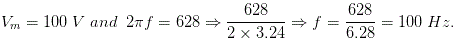05․ The value of supply voltage for 400 W, 4 Ω load is
40 V.
400 V.
20 V.
200 V.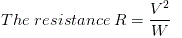Where, V is supply voltage and W is wattage rating of the lamp. Here, W = 400 Watts and R = 4 Ω.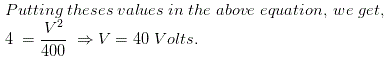06․ Say A point has an absolute potential of 40 V and point B has an absolute potential of - 10 V, then what will be the value of VBA ?
- 50 V.
50 V.
30 V.
none of above.

VBA is defined as VB - VA = -10 - 40 = - 50 V.

07․ The rms value of the voltage U(t)= 3 + 4cos(3t)
5 V.
4.123 V.
7 V.
{3 + 2(1.141)} V.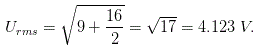08․ In the figure, the potential difference between points P and Q is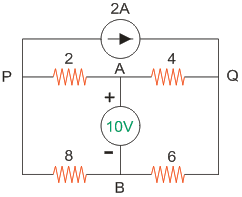6 V.
- 6 V.
10 V.
12 V.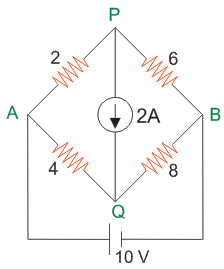Applying KCL at P, VP = 4.8V Applying KCL at Q, VQ = 10.8V Therefore, potential difference between P and Q is 4.8V-10.8V = -6V.

09․ A coil of negligible resistance has an induction of 100 mH. The current passing through the coil changes from 2 A to 4 A at a uniform rate in 0.1 sec the voltage across the coil during this time would be _____________ V.
2.
8.
36.
50.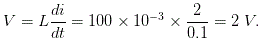10․ What is represented by the hypotenuse of impedance triangle?
Impedance drop.
Resistance drop.
Reactance drop.
Apparent power.

Impedance triangle means the right angle triangle formed by the vectors representing the resistance drop, reactance drop and the impedance drop of the circuit carrying an alternating current.

<<<3839404142>>>